## Selina Concise Mathematics Class 10 ICSE Solutions Chapter 1 Value Added Tax Ex 1B

These Solutions are part of Selina Concise Mathematics Class 10 ICSE Solutions. Here we have given Selina Concise Mathematics Class 10 ICSE Solutions Chapter 1 Value Added Tax Ex 1B.

Other Exercises

[In this exercise, all the prices are excluding tax/VAT unless specified].
Question 1.
A shopkeeper purchases an article for ₹ 6,200 and sells it to a customer for ₹ 8,500. If the sale tax (under VAT) is 8%; find the VAT paid by the shopkeeper.
Solution:
C.P. of article = ₹ 6200
Rate of VAT = 8%Amount of VAT paid by the shopkeeper = ₹ 680 – ₹ 496 = ₹ 184

Question 2.
A purchases an article for ₹ 3,600 and sells it to B for ₹ 4,800. B, in turn, sells the article to C for ₹ 5,500. If the sale tax (under VAT) is 10%, find the VAT levied on A and B.
Solution:
C.P. of an article for A = ₹ 3600
C.P. of the article for B = ₹ 4800
and C.P. for C = ₹ 5500
Rate of VAT in each case = 10%Now VAT levied on A = ₹ 480 – ₹ 360 = ₹ 120
and VAT levied on B = ₹ 550 – ₹ 480 = ₹ 70

Question 3.
A manufacturer buys raw material for ₹ 60,000 and pays 4% tax. He sells the ready stock for ₹ 92,000 and charges 12.5% tax. Find the VAT paid by the manufacturer.
Solution:
C.P. of raw material = ₹ 60000
Rate of tax = 4%VAT paid by the manufacturer = ₹ 11500 – ₹ 2400 = ₹ 9100

Question 4.
The cost of an article is ₹ 6,000 to a distributor. He sells it to a trader for ₹ 7,500 and the trader sells it to a customer for ₹ 8,000. If the VAT rate is 12.5% ; find the VAT paid by the :
(i) distributor
Solution:
Cost price of an article to a distributor = ₹ 6000
and selling price of distributor = ₹ 7500
and selling price of trader = ₹ 8000
Rate of VAT = 12.5% = $$\frac { 25 }{ 2 }$$ %
Now, VAT for two distributor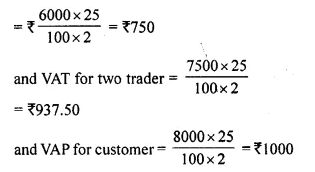(i) Now VAT paid by distributor = ₹ 937.50 – ₹ 750 = ₹ 187.50
(ii) and VAT paid by trader = ₹ 1000 – ₹ 937.50 = ₹ 62.50

Question 5.
The printed price of an article is ₹ 2,500. A wholesaler sells it to a retailer at 20% discount and charges sales-tax at the rate of 10%. Now the retailer, in turn, sells the article to a customer at its list price and charges the sales-tax at the same rate. Find :
(i) the amount that retailer pays to the wholesaler.
(ii) the VAT paid by the retailer.
Solution:
M.P. of an article = ₹ 2500
Rebate = 20%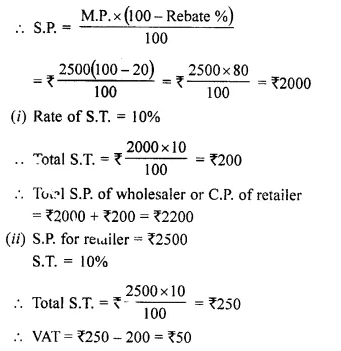Question 6.
A retailer buys an article for ₹ 800 and pays the sales-tax at the rate of 8%. The retailer sells the same article to a customer for ₹ 1,000 and charges sales- tax at the same rate. Find :
(ii) the amount of VAT paid by the retailer.
Solution:
C.P. of an article for retailer = ₹ 800
Rate of S.T. = 8%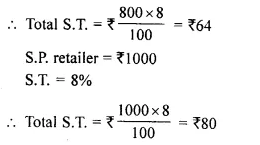(i) Cost price of the customer = ₹ 1000 + ₹ 80 = ₹ 1080
(ii) VAT paid by the retailer = ₹ 80 – ₹ 64 = ₹ 16

Question 7.
A shopkeeper buys 15 identical articles for ₹ 840 and pays sales-tax at the rate of 8%. He sells 6 of these articles at ₹ 65 each and charges sales-tax at the same rate. Calculate the VAT paid by the shopkeeper against the sale of these six articles.
Solution:
C.P. of 15 articles = ₹ 840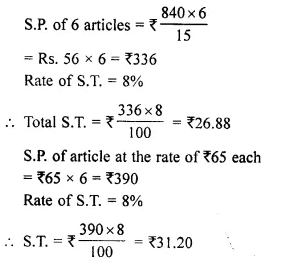VAT paid by the retailer = ₹ 31.20 – ₹ 26.88 = ₹ 4.32

Question 8.
The marked price of an article is ₹ 900 and the rate of sales-tax on it is 6%. If on selling the article at its marked price, a retailer has to pay VAT = ₹ 4.80; find the money paid by him (including sales-tax) for purchasing this article.
Solution:
M.P. an article = ₹ 900
Rate of S.T. = 6%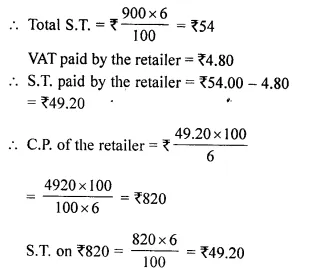Amount paid including S.T. = ₹ 820 + ₹ 49.20 = ₹ 869.20

Question 9.
A manufacturer marks an article at ₹ 5,000. He sells this article to a wholesaler at a discount of 25% on the marked price and the wholesaler sells it to a retailer at a discount of 15% on its marked price. If the retailer sells the article without any discount and at each stage the sales-tax is 8%, calculate the amount of VAT paid by :
(i) the wholesaler.
(ii) the retailer.
Solution:
M.P. of an article = ₹ 5000
Rate of discount = 25%
S.R of the manufacturer or C.P of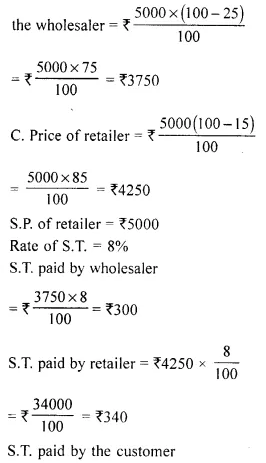Now VAT paid by
(i) The wholesaler = ₹ 340 – ₹ 300 = ₹ 40
(ii) Retailer = ₹ 400 – ₹ 340 = ₹ 60

Question 10.
A shopkeeper buys an article at a discount of 30% and pays sales-tax at the rate of 8%. The shopkeeper, in turn, sells the article to a customer at the printed price and charges sales tax at the same rate. If the printed price of the article is ₹ 2,500; find :
(i) the price paid by the shopkeeper.
(ii) the price paid by the customer.
(iii) The VAT (Value Added Tax) paid by the shopkeeper.
Solution:
Printed price of an article = ₹ 2500
Discount = 30%
C.P of the article for shopkeeper(i) Price paid by the shopkeeper = ₹ 1750 + ₹ 140 = ₹ 1890
(ii) Price paid by the customer = ₹ 2500 + ₹ 200 = ₹ 2700
(iii) VAT paid by the shopkeeper = ₹ 200 – ₹ 140 = ₹ 60

Question 11.
A shopkeeper sells an article at its list price (₹ 3,000) and charges sales-tax at the rate of 12%. If the VAT paid by the shopkeeper is ₹ 72, at what price did the shopkeeper buy the article inclusive of sales-tax ?
Solution:
S.P. or list price of an article = ₹ 3000
Rate of sales tax = 12%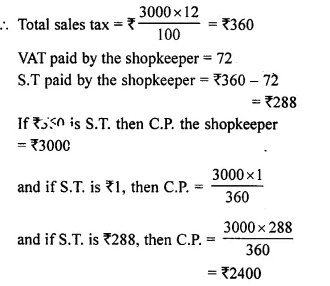C.P paid by the shopkeeper including S.T. = ₹ 2400 + ₹ 288 = ₹ 2688

Question 12.
A manufacturer marks an article for ₹ 10,000. He sells it to a wholesaler at 40% discount. The wholesaler sells this article to a retailer at 20% discount on the marked price of the article. If retailer sells the article to a customer at 10% discount and the rate of sales-tax is 12% at each stage; find the amount of VAT paid by the :
(i) wholesaler
(ii) retailer
Solution:
Marked price of an article = ₹ 10000
Rate of discount = 40%
C.P of the wholesalerNow
(i) Vat paid by wholesaler = ₹ 960 – ₹ 720 = ₹ 240
(ii) Vat paid by retailer = ₹ 1080 – ₹ 960 = ₹ 120

Hope given Selina Concise Mathematics Class 10 ICSE Solutions Chapter 1 Value Added Tax Ex 1B are helpful to complete your math homework.

If you have any doubts, please comment below. Learn Insta try to provide online math tutoring for you.